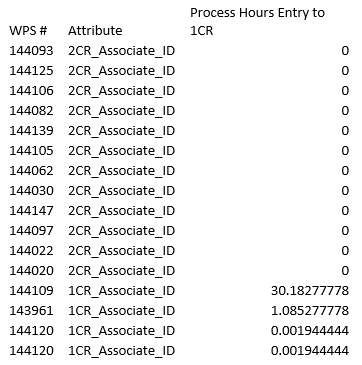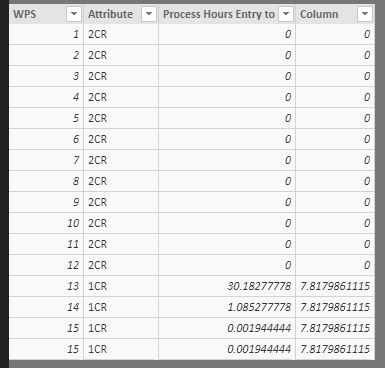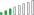cancel
Showing results for
Did you mean:

Fabric is Generally Available. Browse Fabric Presentations. Work towards your Fabric certification with the Cloud Skills Challenge.Frequent Visitor

## Calculate Average with Filter

Good afternoon,

I am trying to create an average using a filter.  In my screen shot below, you can see that under the Attribute column there are two different values.  I want to be able to sum up the Process Hours Entry to 1CR column and divide it by the count of the Attribute column where the value is 1CR_Associate_ID.  Currently when I try and just use the average function it is dividing the total by 16.  Any ideas on how to accomplish this???  Thanks in Advance!!

Renee1 ACCEPTED SOLUTIONCommunity Support

HI, @Rebender

You may try to this formula to create a measure as below:

```Measure =
DIVIDE (
CALCULATE ( SUM ( 'Table'[Process Hours Entry to] ) ),
CALCULATE (
COUNTA ( 'Table'[Attribute] ),
'Table'[Process Hours Entry to] <> 0
),
0
)```

or use this formula to create a column

```Column = DIVIDE (
CALCULATE ( SUM ( 'Table'[Process Hours Entry to] ),FILTER('Table','Table'[Attribute]=EARLIER('Table'[Attribute]) )),
CALCULATE (
COUNTA ( 'Table'[Attribute] ),FILTER('Table','Table'[Attribute]=EARLIER('Table'[Attribute])&&
'Table'[Process Hours Entry to] <> 0)
),
0
)```

Result:measurecolumn

here is pbix, please try it.

https://www.dropbox.com/s/rgvy7m8w1l15we6/Calculate%20Average%20with%20Filter.pbix?dl=0

Best Regards,

Lin

Community Support Team _ Lin
If this post helps, then please consider Accept it as the solution to help the other members find it more quickly.
10 REPLIES 10Is there anything in Power BI that isn't ridiculously difficult to do?Community Support

HI, @Rebender

You may try to this formula to create a measure as below:

```Measure =
DIVIDE (
CALCULATE ( SUM ( 'Table'[Process Hours Entry to] ) ),
CALCULATE (
COUNTA ( 'Table'[Attribute] ),
'Table'[Process Hours Entry to] <> 0
),
0
)```

or use this formula to create a column

```Column = DIVIDE (
CALCULATE ( SUM ( 'Table'[Process Hours Entry to] ),FILTER('Table','Table'[Attribute]=EARLIER('Table'[Attribute]) )),
CALCULATE (
COUNTA ( 'Table'[Attribute] ),FILTER('Table','Table'[Attribute]=EARLIER('Table'[Attribute])&&
'Table'[Process Hours Entry to] <> 0)
),
0
)```

Result:measurecolumn

here is pbix, please try it.

https://www.dropbox.com/s/rgvy7m8w1l15we6/Calculate%20Average%20with%20Filter.pbix?dl=0

Best Regards,

Lin

Community Support Team _ Lin
If this post helps, then please consider Accept it as the solution to help the other members find it more quickly.Frequent Visitor

Thank you so much for your help!  I have just one more question....  How would I change the code if I want to have this divide by a distinct count of WPS?  I have two rows that have the same number so I would like to sum the 4 rows hours and then divide by a distinct count of WPS (3)...

Thanks again!

ReneeResolver III

Helle @Rebender,

Try this;

```Measure2 =
DIVIDE (
CALCULATE ( SUM ( 'Table'[Process Hours Entry to] ) );
CALCULATE (
DISTINCTCOUNT( ( 'Table'[WPS] ));
'Table'[Process Hours Entry to] <> 0
);
0
)```

Greets,

RonaldFrequent Visitor

This is working great except I have one more question/problem.....  I have slicers on my report that the measure seems to be ignoring.   Do I have to do something else in order for the measure to use the slicers?

thanks again!!!

ReneeCommunity Support

hi, @Rebender

You should check these slicers if had created the relationship with this table and the cross filter direction if is both.

otherwise it should can be affected by slicer.

Best Regards,

Lin

Community Support Team _ Lin
If this post helps, then please consider Accept it as the solution to help the other members find it more quickly.Resolver III

Hello @Rebender,

If the date field in the slicer is from an another table, you must make a connection between the differt tables.
Otherwise, you must give more information because a slicer with a data field from the table of your'e measures must work,

Greets,

RonaldResolver III

Hello @Rebender,

Try this;

```AverageFilter = DIVIDE(
SUM(Table1[1CR]);
CALCULATE(
COUNT(Table1[Attribute]);
Table1[Attribute]="1CR_Associate_ID"))```

Greets,

RonaldSuper User

Perhaps something along the lines of:

```Measure =
VAR __attribute = MAX('Table'[Attribute])
RETURN AVERAGEX(FILTER('Table',[Attribute]=__attribute),[1CR])```

Put in table visual along with Attribute.

Become an expert!: Enterprise DNA
External Tools: MSHGQM
Latest book!:
Mastering Power BI 2nd EditionDAX is easy, CALCULATE makes DAX hard...Frequent Visitor

Thanks for you assistance!  Any idea why the value calculates out to 8.17?  If I add the numbers and divide by 4 I come up with 7.81....Announcements#### Power BI Monthly Update - November 2023

Check out the November 2023 Power BI update to learn about new features.#### Fabric Community News unified experience

Read the latest Fabric Community announcements, including updates on Power BI, Synapse, Data Factory and Data Activator.#### The largest Power BI and Fabric virtual conference

130+ sessions, 130+ speakers, Product managers, MVPs, and experts. All about Power BI and Fabric. Attend online or watch the recordings.Top Solution Authors
Top Kudoed Authors
Users online (2,900)